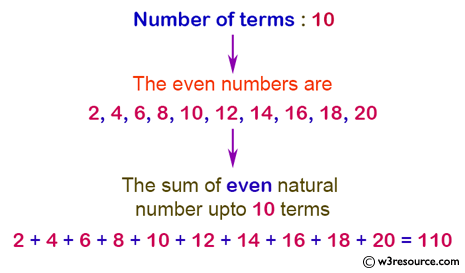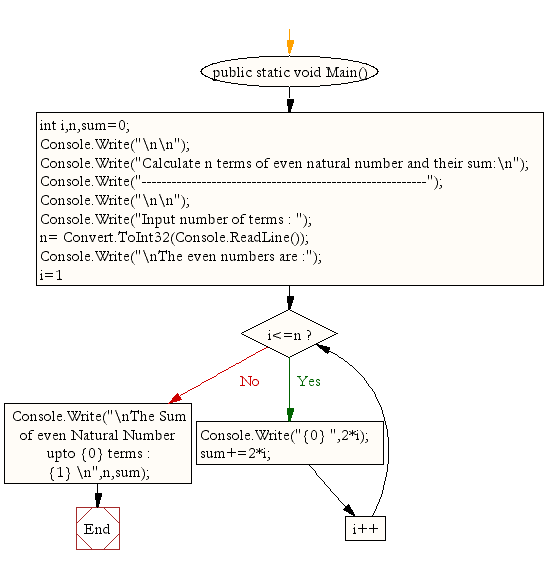﻿ C Sharp - Find n terms of even natural number and their sum# C# Sharp Exercises: Calculate n terms of even natural number and their sum

## C# Sharp For Loop: Exercise-16 with Solution

Write a program in C# Sharp to display the n terms of even natural number and their sum.

Pictorial Presentation:Sample Solution:

C# Sharp Code:

``````using System;
public class Exercise16
{
public static void Main()
{
int i,n,sum=0;

Console.Write("\n\n");
Console.Write("Calculate n terms of even natural number and their sum:\n");
Console.Write("---------------------------------------------------------");
Console.Write("\n\n");

Console.Write("Input number of terms : ");
Console.Write("\nThe even numbers are :");
for(i=1;i<=n;i++)
{
Console.Write("{0} ",2*i);
sum+=2*i;
}
Console.Write("\nThe Sum of even Natural Number upto {0} terms : {1} \n",n,sum);
}
}
```
```

Sample Output:

```Calculate n terms of even natural number and their sum:
---------------------------------------------------------
Input number of terms : 10
The even numbers are :2 4 6 8 10 12 14 16 18 20
The Sum of even Natural Number upto 10 terms : 110
```

Flowchart:C# Sharp Code Editor:

Contribute your code and comments through Disqus.

What is the difficulty level of this exercise?

Test your Programming skills with w3resource's quiz.

﻿